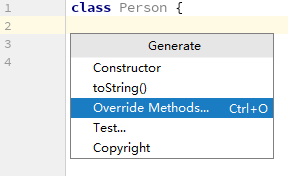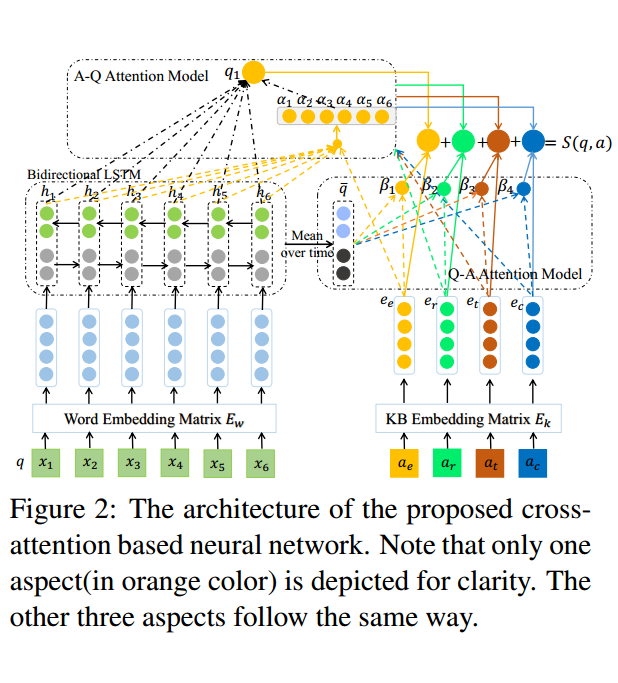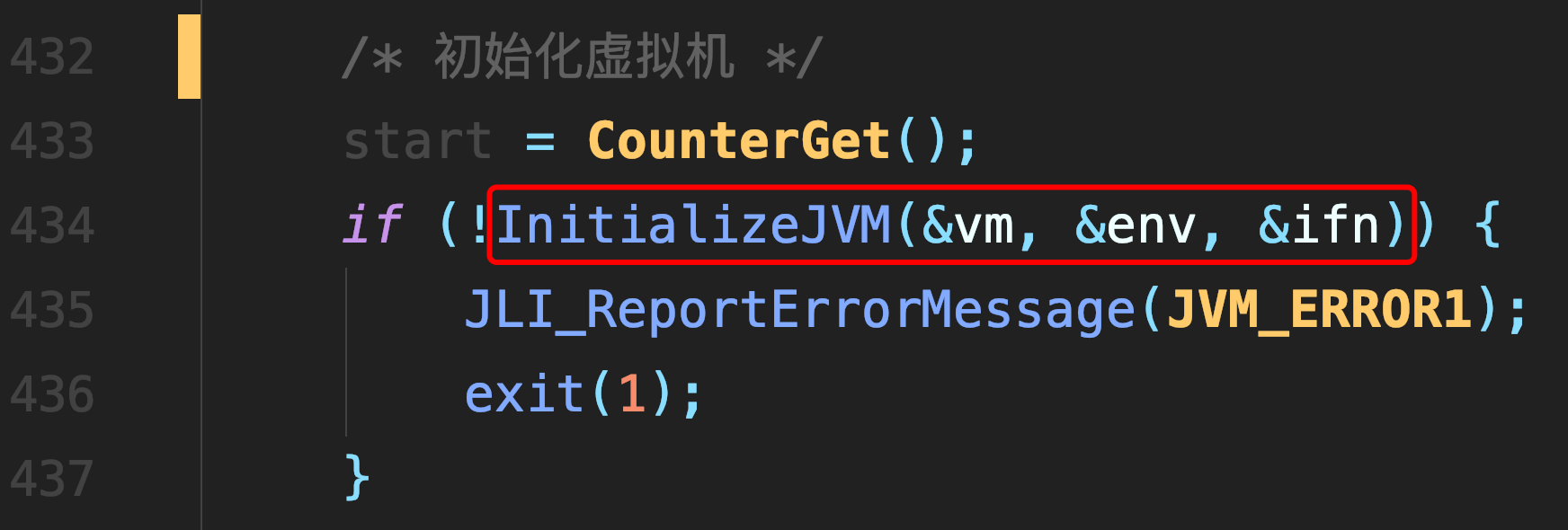# 1003. Emergency (25)

400 ms

65536 kB

16000 B

Standard

CHEN, Yue

As an emergency rescue team leader of a city, you are given a special map of your country. The map shows several scattered cities connected by some roads. Amount of rescue teams in each city and the length of each road between any pair of cities are marked on the map. When there is an emergency call to you from some other city, your job is to lead your men to the place as quickly as possible, and at the mean time, call up as many hands on the way as possible.

Input

Each input file contains one test case. For each test case, the first line contains 4 positive integers: N (<= 500) - the number of cities (and the cities are numbered from 0 to N-1), M - the number of roads, C1 and C2 - the cities that you are currently in and that you must save, respectively. The next line contains N integers, where the i-th integer is the number of rescue teams in the i-th city. Then M lines follow, each describes a road with three integers c1, c2 and L, which are the pair of cities connected by a road and the length of that road, respectively. It is guaranteed that there exists at least one path from C1 to C2.

Output

For each test case, print in one line two numbers: the number of different shortest paths between C1 and C2, and the maximum amount of rescue teams you can possibly gather.
All the numbers in a line must be separated by exactly one space, and there is no extra space allowed at the end of a line.

Sample Input

5 6 0 2
1 2 1 5 3
0 1 1
0 2 2
0 3 1
1 2 1
2 4 1
3 4 1

Sample output

2 4

``````#include<iostream>
using namespace std;
#include<cstdio>
#include<map>
#include<vector>
const int I_NF = 501;
const int MAX = 0x7FFFFF;
int n, m, c1, c2; //n为城市数， m为road数， c1, c2 始发地 目的地
int group[I_NF];
bool flag[I_NF];
map<int, vector<pair<int, int> > > M; //迭代器的使用

int mDistance = MAX; //最短距离
int maNumber = 0; //最大的救援队
int teams; //不同的路径有多少
int DFS(int start, int distance, int number) //在DFS的过程中每次都会更新着三个参数
{
if(start == c2)
{
if(distance == mDistance)
{
if(number > maNumber)
maNumber = number;
teams++;
}
else if(distance < mDistance)
{
teams = 1;
mDistance = distance;
maNumber = number;
}
return 0;
}

flag[start] = true;  //标记
vector<pair<int, int> >::iterator it;
if(M.count(start))
{
for(it = M[start].begin(); it != M[start].end(); it++)
{
if(!flag[it->first])  DFS(it->first, distance+it->second, number+group[it->first]);
}
}
flag[start] = false; //释放标记
return 0;
}

int main()
{
//freopen("E:/in.txt", "r", stdin);
scanf("%d%d%d%d", &n, &m, &c1, &c2);
for(int i = 0; i < n; i++)
{
scanf("%d", &group[i]);
}

int start, end, distance;
for(int i = 0; i < m; i++)
{
scanf("%d%d%d", &start, &end, &distance);
M[start].push_back(make_pair(end, distance));
M[end].push_back(make_pair(start, distance));//注意是无向图
}

for(int i = 0; i < n; i++)  flag[i] = false;

DFS(c1, 0, group[c1]);

printf("%d %d\n", teams, maNumber);
return 0;
} ``````

##### 热门推荐
•浏览(655)
•浏览(565)
•浏览(548)
•浏览(488)
•浏览(434)
•浏览(424)
•浏览(381)
•浏览(367)
•浏览(362)
•浏览(357)